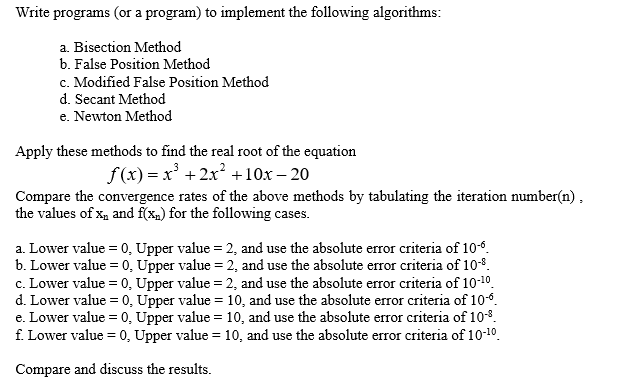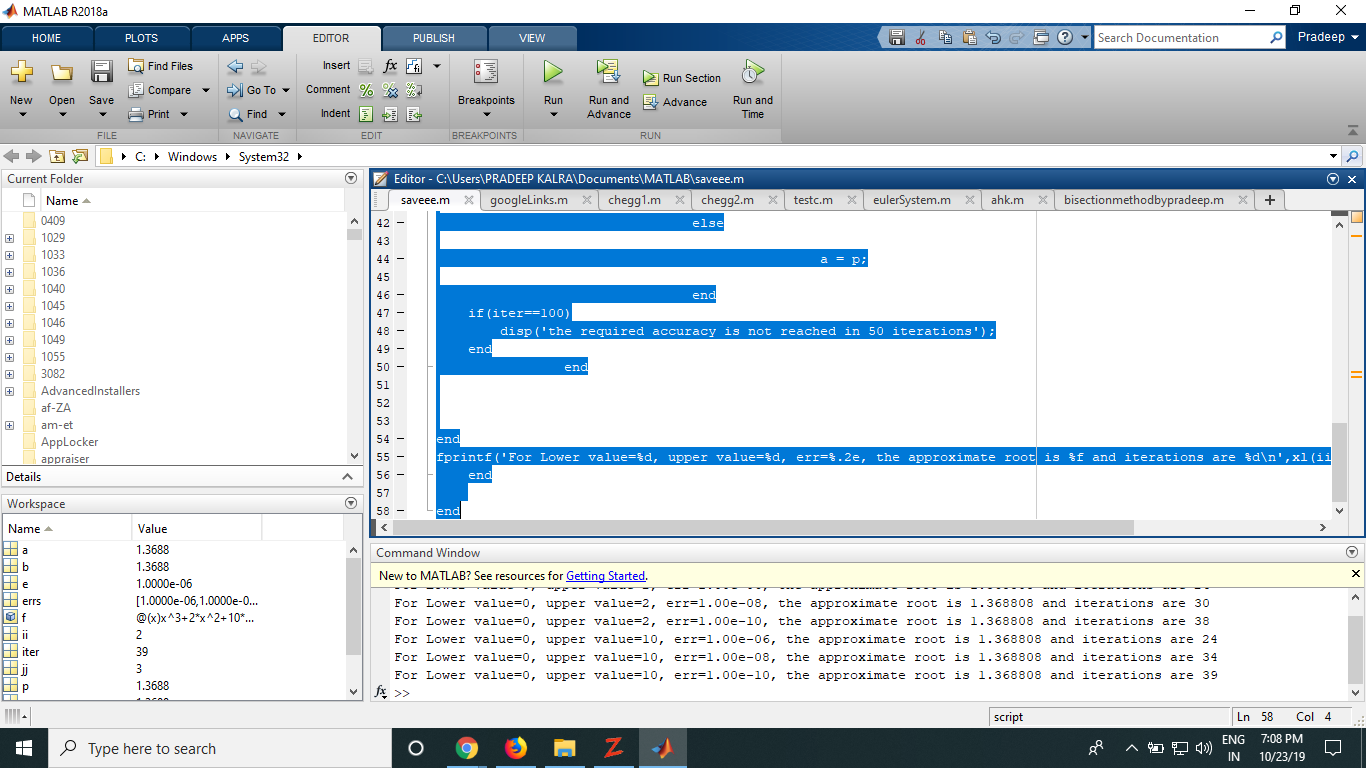# (Solved) : Needed Matlab Solution Got Answer Need 1 Short Summary Method Work 2 Plots Demonstrate Re Q41384300 . . .I needed a Matlab solution so I got this as an answer and now Ido need this.

1)Short summary of how method work.

2) Plots that demonstrate your results, e.g. iteration vs. x,iteration vs. f(x) and iteration vs. error.

3) Do not tabulate results of the all iterations, usegraphs.

4)Discuss what is happening when you reduce the tolerance andchange the intervals.

Solution:

a)

Bisectionmethod

clc
clear all
close all
format long
xl=[0,0];
xu=[2,10];
errs=[1e-6,1e-8,1e-10];
for ii=1:length(xl)
for jj=1:length(errs)
f = @(x) x^3+2*x^2+10*x-20;
e=1e-6;
a=xl(ii);
b=xu(ii);
iter = 0;

if f(a)*f(b)>=0

disp(‘No Root’)

else

prev = (a+b)/2;
p=a;
while (abs(f(p))>errs(jj))
prev=p;

iter =iter+ 1;

p = (a+b)/2;

if f(p) == 0
p
q=1
break;

end

if f(a)*f(p)<0

b = p;

else

a = p;

end
if(iter==100)
disp(‘the required accuracy is not reached in 50iterations’);
end
end

end
fprintf(‘For Lower value=%d, upper value=%d, err=%.2e, theapproximate root is %f and iterations are%dn’,xl(ii),xu(ii),errs(jj),p,iter);
end

endWe were unable to transcribe this image- O X Pradeep VIEW do E C . Search Documentation D EDITOR PUBLISH Insert E fx EH – Comment % 92% Breakpoints Indent & EDIT Run Section 8 Run Run and Advance Advance Aduan RUN Run and Time BREAKPOINTS O ох Editor – C:UsersPRADEEP KALRADocumentsMATLABsaveee.m saveee. mx googleLinks. mx chegg1. mx chegg2. mx else testcm x eulerSystem. m x ahk. mx bisectionmethodbypradeep.m x + 1 MATLAB R2018a HOME PLOTS APPS + G C Find Files 3 Compare – Go To – New Open Save Print Find FILE NAVIGATE a Windows System32 Current Folder Name 0409 1029 1033 1036 1040 1045 1046 1049 1055 3082 Advancedinstallers af-ZA am-et AppLocker appraiser Details a = p; L L LLLLL end if(iter==100) disp(‘the required accuracy is not reached in 50 iterations) end end end fprintf(‘For Lower value=&d, upper value=\$d, err=\$.2e, the approximate root is \$f and iterations are \$dn’,xl(ii end < O Workspace end Name b Value 1.3688 1.3688 1.0000e-06 [1.0000e-06, 1.0000e-… @(x)x^3+2*x^2+10* … Command Window New to MATLAB? See resources for Getting Started. H errs For Lower value=0, upper value=2, err=1.00e-08, the approximate root is 1.368808 and iterations are 30 For Lower value=0, upper value=2, err=1.00e-10, the approximate root is 1.368808 and iterations are 38 For Lower value=0, upper value=10, err=1.00e-06, the approximate root is 1.368808 and iterations are 24 For Lower value=0, upper value=10, err=1.00e-08, the approximate root is 1.368808 and iterations are 34 For Lower value=0, upper value=10, err=1.00e-10, the approximate root is 1.368808 and iterations are 39 39 1.3688 script O9 Type here to search In 58 Col 4 ENG 7:08 PM 0 IN 10/23/19 Z- ” Show transcribed image text
– O X Pradeep VIEW do E C . Search Documentation D EDITOR PUBLISH Insert E fx EH – Comment % 92% Breakpoints Indent & EDIT Run Section 8 Run Run and Advance Advance Aduan RUN Run and Time BREAKPOINTS O ох Editor – C:UsersPRADEEP KALRADocumentsMATLABsaveee.m saveee. mx googleLinks. mx chegg1. mx chegg2. mx else testcm x eulerSystem. m x ahk. mx bisectionmethodbypradeep.m x + 1 MATLAB R2018a HOME PLOTS APPS + G C Find Files 3 Compare – Go To – New Open Save Print Find FILE NAVIGATE a Windows System32 Current Folder Name 0409 1029 1033 1036 1040 1045 1046 1049 1055 3082 Advancedinstallers af-ZA am-et AppLocker appraiser Details a = p; L L LLLLL end if(iter==100) disp(‘the required accuracy is not reached in 50 iterations) end end end fprintf(‘For Lower value=&d, upper value=\$d, err=\$.2e, the approximate root is \$f and iterations are \$dn’,xl(ii end